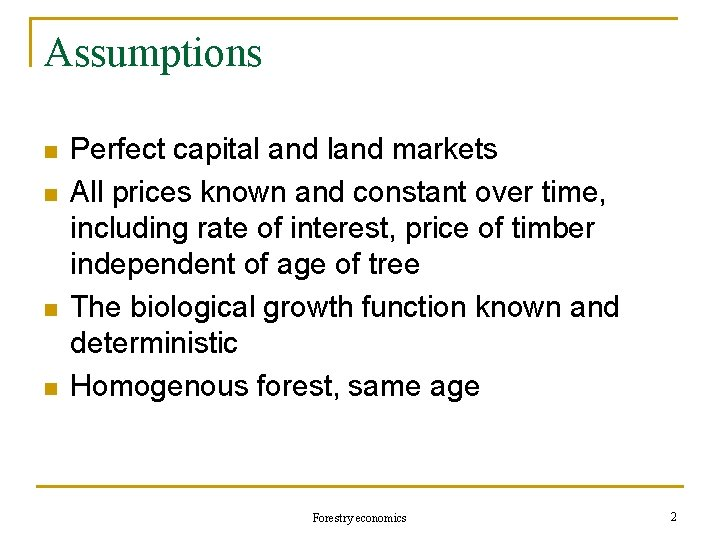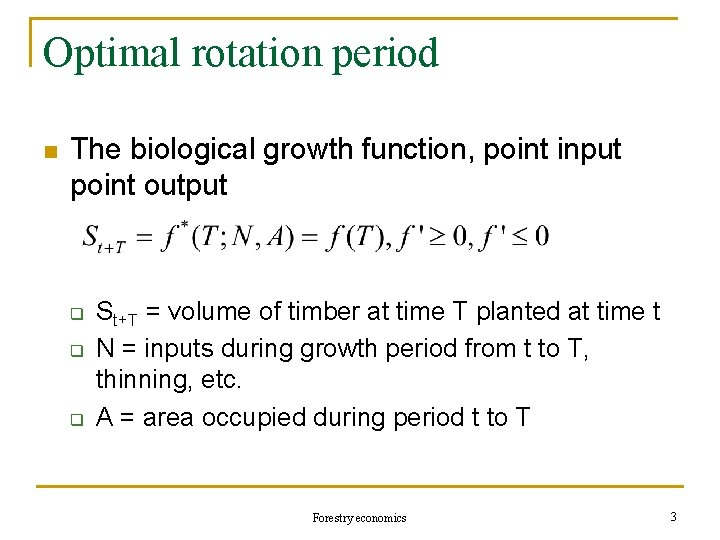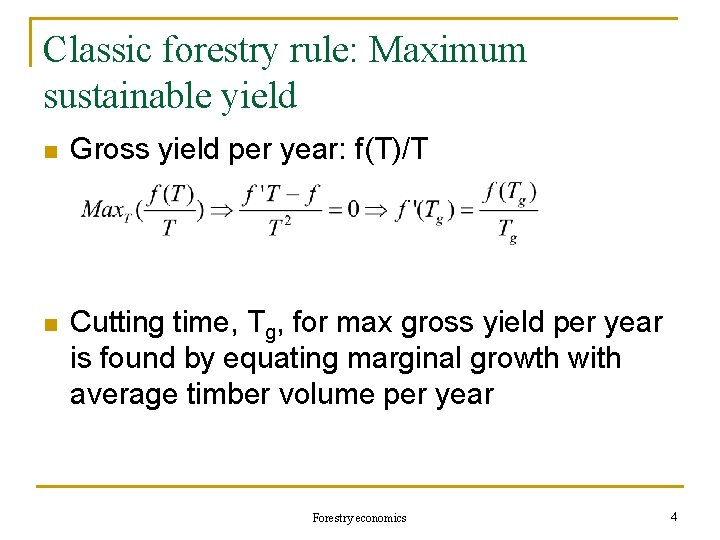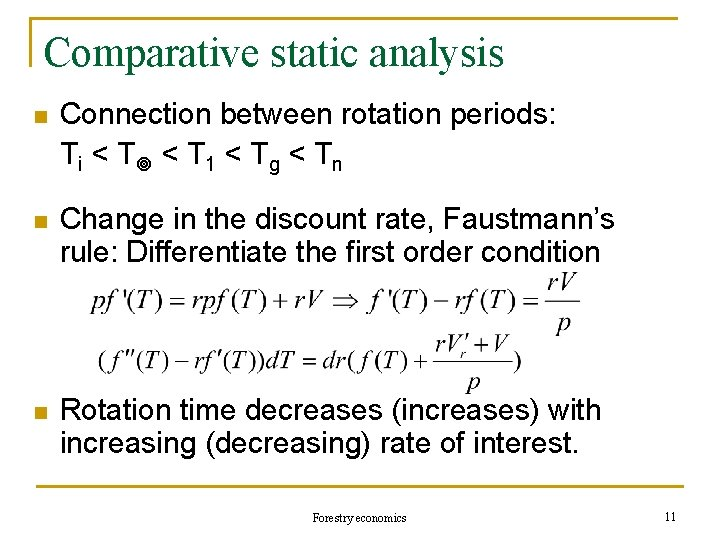# ECON 4925 Autumn 2006 Resource Economics Forestry economics

• Slides: 11ECON 4925 Autumn 2006 Resource Economics Forestry economics Lecturer: Finn R. Førsund Forestry economics 1Assumptions n n Perfect capital and land markets All prices known and constant over time, including rate of interest, price of timber independent of age of tree The biological growth function known and deterministic Homogenous forest, same age Forestry economics 2Optimal rotation period n The biological growth function, point input point output q q q St+T = volume of timber at time T planted at time t N = inputs during growth period from t to T, thinning, etc. A = area occupied during period t to T Forestry economics 3Classic forestry rule: Maximum sustainable yield n Gross yield per year: f(T)/T n Cutting time, Tg, for max gross yield per year is found by equating marginal growth with average timber volume per year Forestry economics 4Maximum sustainable yield, cont. n Net yield per year: (pf(T) - k) / T, k= planting costs, price p net of cutting costs n Cutting time, Tn, for max net yield per year is found by equating marginal growth with average timber volume per year subtracted average wages measured in timber. Forestry economics 5One rotation period: Jevon’s rule n Cutting time, T 1, for max one period yield is found by equating marginal growth with rate of interest multiplied with total timber volume, i. e. marginal growth equal to the interest on felling timber measured in timber units. Forestry economics 6Internal rate of return n Definition of internal rate of return n Maximisation of internal rate of return Forestry economics 7Faustmann’s rule n Present value of ongoing timber production, i. e. replanting on the same land to infinity n Sum of infinite geometric series Forestry economics 8Faustmann’s rule, cont. n Maximisation of present value, V, of rotation cycles to infinity Forestry economics 9Interpretation of Faustmann’s rule Value of marginal growth equal to interest on value of timber plus interest on value of land (i. e. rental land value) Growth rate greater than the rate of interest Forestry economics 10Comparative static analysis n Connection between rotation periods: Ti < T 1 < Tg < Tn n Change in the discount rate, Faustmann’s rule: Differentiate the first order condition n Rotation time decreases (increases) with increasing (decreasing) rate of interest. Forestry economics 11